# MMA图形变换分析

### 1.分式线性变换

${\rm{TransformationFunction}}\left[ {\left( {\begin{array}{*{20}{c}} 1&2&5\\ 3&4&6\\ 7&8&1 \end{array}} \right)} \right]$

$\left\{ {x,y} \right\} \to \left\{ {\frac{{5 + x + 2y}}{{1 + 7x + 8y}},\frac{{6 + 3x + 4y}}{{1 + 7x + 8y}}} \right\}$

$\left\{ {x,y} \right\} \to \left\{ {x + 2y,3x + 4y} \right\}$

$\left[ {\begin{array}{*{20}{c}} 1&2\\ 3&4 \end{array}} \right]\left[ {\begin{array}{*{20}{c}} {{x_n}}\\ {{y_n}} \end{array}} \right] = \left[ {\begin{array}{*{20}{c}} {{x_m}}\\ {{y_m}} \end{array}} \right]$

### 2.简单例子

$\begin{array}{*{20}{l}} {\left[ {\begin{array}{*{20}{c}} a&b\\ c&d \end{array}} \right]\left[ {\begin{array}{*{20}{c}} 0\\ 1 \end{array}} \right] = \left[ {\begin{array}{*{20}{c}} b\\ d \end{array}} \right]}\\ {\left[ {\begin{array}{*{20}{c}} a&b\\ c&d \end{array}} \right]\left[ {\begin{array}{*{20}{c}} 1\\ 0 \end{array}} \right] = \left[ {\begin{array}{*{20}{c}} a\\ c \end{array}} \right]} \end{array}$

$\left[ {\begin{array}{*{20}{c}} a&c\\ b&d \end{array}} \right]$

### 3.逆矩阵获得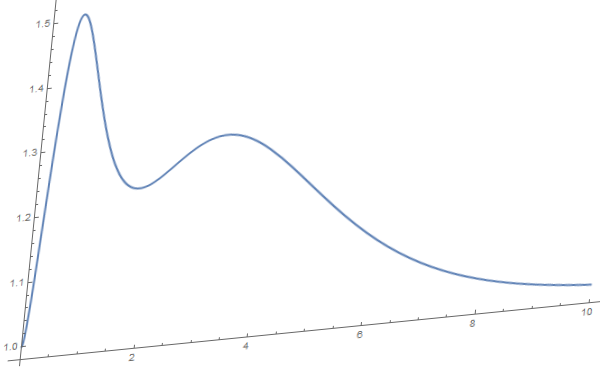MMA图形变换分析

$\left[ {\begin{array}{*{20}{c}} 1&{0.1}\\ {0.1}&1 \end{array}} \right]\left[ {\begin{array}{*{20}{c}} {{x_n}}\\ {{y_n}} \end{array}} \right] = \left[ {\begin{array}{*{20}{c}} {{x_m}}\\ {{y_m}} \end{array}} \right]$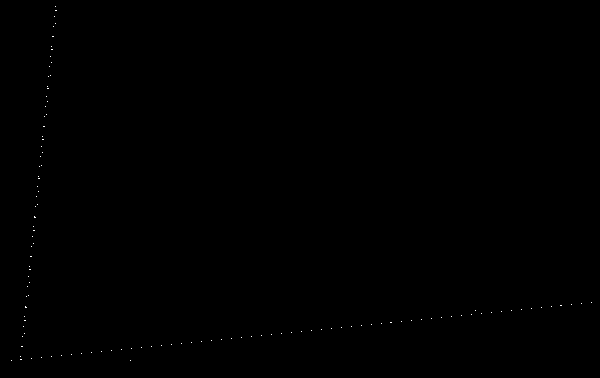MMA图形变换分析

$\left[ {\begin{array}{*{20}{c}} {600.}&{59.92}\\ { – 38.51}&{ – 378.} \end{array}} \right]$

$\left[ {\begin{array}{*{20}{c}} 1&{0.1}\\ {0.1}&1 \end{array}} \right] \Leftrightarrow \left[ {\begin{array}{*{20}{c}} {600.}&{59.92}\\ { – 38.51}&{ – 378.} \end{array}} \right]$

$\left[ {\begin{array}{*{20}{c}} {1.01}&{ – 0.10}\\ { – 0.10}&{1.01} \end{array}} \right]$

### 4.图像处理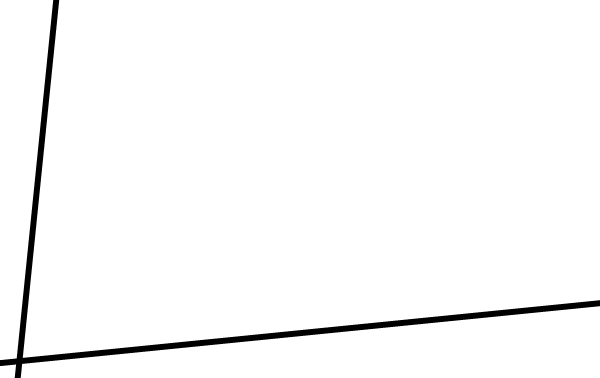MMA图形变换分析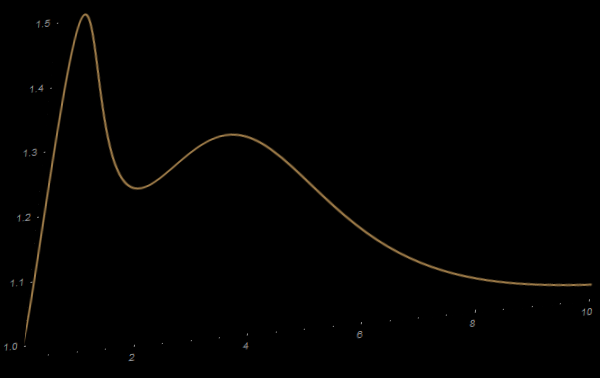MMA图形变换分析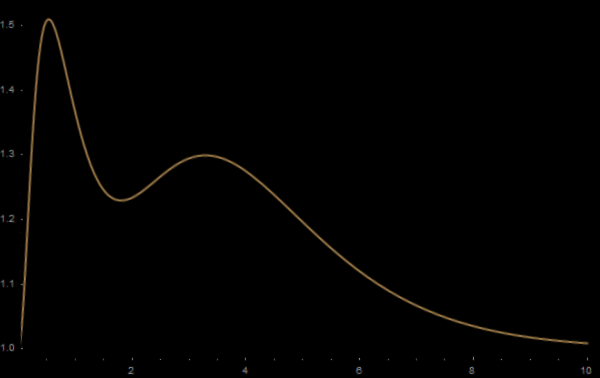MMA图形变换分析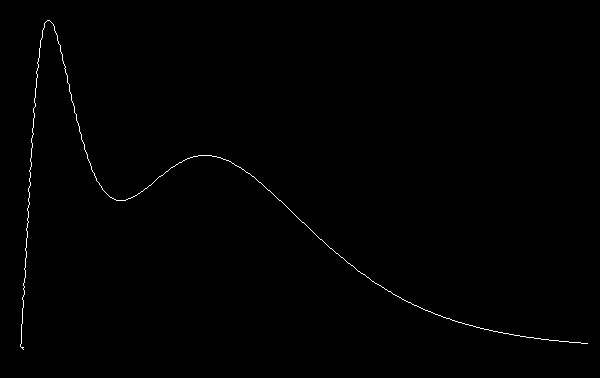MMA图形变换分析

### 5.End小结

Anyway，总算是晕晕乎乎的写完了，也不知道其中有多少问题。这个东西还是蛮实用的，所以就留下来以供以后参考参考。

### 6.Reference